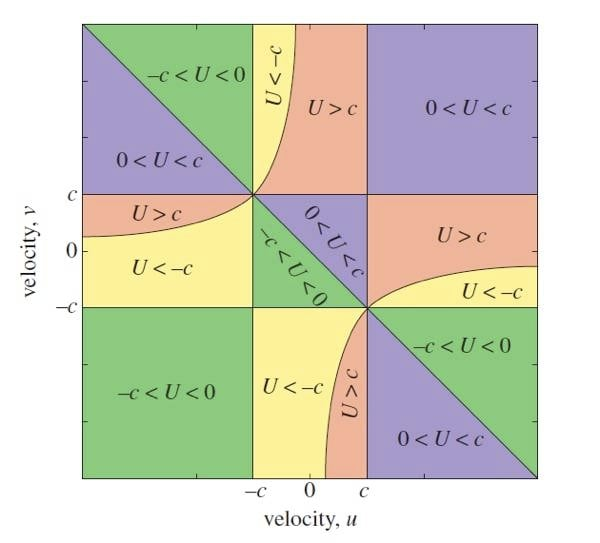# Is lightspeed really a limit?

## Solving super-luminal Special Relativity without breaking Einstein

We don’t (yet) have any way to test this, but University of Adelaide applied mathematicians are suggesting that an extended version of Einstein’s Theory of Special Relativity also holds true for velocities beyond lightspeed.

One of the main predictions of Special Relativity is that the speed of light is treated as an absolute cosmic speed limit, the line which can never be crossed; and even the notorious “faster-than-light neutrino” incident in 2011 has left the theory intact as one of the most robust in physics.

However, during the speculation that surrounded the neutrino discussion last year1, the University of Adelaide’s Professor Jim Hill and Dr Barry Cox considered the question of how the mathematical contradictions posed by a faster-than-light particle could be aligned with Special Relativity.

Their solution, which Professor Hill discussed with The Register,2 rested on ignoring the speed of light’s status as an absolute limit, and instead, using the information where the relative velocity of two observers is infinite.3.Outside the box: Einstein's Special Relativity works inside the smallest square.

The University of Adelaide researchers have extended the mathematics

to a world beyond Einstein's limit. Image provided by Professor Jim Hill

The surprising outcome: with just two assumptions, an extended version of the mathematics for Einstein's special relativity works just as well above the speed of light as below.

“Relativity is about frames of reference,” Professor Hill explained to The Register. That is, observers with different velocities see the same event from different frames of reference.

“Einstein started working from information where the relative velocity is zero – what we knew about, such as rest mass, kinetic energy and so on – and then extrapolated what is known in the Newtonian world for velocities lower than c.

“Our thinking was: how do we make use of the essential essence of Einstein’s theory for velocities above c?”

Mathematically, what the mathematicians assumed is that for infinite relative velocity, there is a fixed relationship between the velocities of the two observers: where u is the first observer’s velocity, v is the second, the product of the two velocities is always c2.

“What we have is an equivalent theory [to Special Relativity] that applies for velocities beyond the speed of light. That theory is different from Special Relativity, but it has many of the same characteristics.

And readers with an interest in either physics or maths will be delighted with the vital assumptions: there has to be one, and only one, speed of light; and in all cases, a mathematical singularity occurs at the speed of light.

“If you believe what we’ve done,” Professor Hill said, “there can only be one speed of light in a universe. If there was a second speed of light, our mathematics wouldn’t work. If there is a second singularity [the one that occurs at the speed of light in Special Relativity – The Register] it wouldn’t work.

“This theory and method of solution is dependent on assuming that there is only one speed of light in any universe.”

To get from the theory to any practical test is another matter entirely, and Professor Hill freely admits he doesn’t know how that might be achieved (although The Register notes that the world took half a century to get from the maths of emission of radiation to the laser). He hopes, however, that a test can be devised.

“If you really don’t believe that faster-than-light is possible, then humans will be limited forever,” he said.

Einstein’s special relativity beyond the speed of light has been published in the Proceedings of the Royal Society. ®

### Bootnotes

1. The faster-than-light neutrino 'discovery' that ended with a cable fault.

2. I freely admit that I was gasping to keep up during this interview. Strike that: I was failing to keep up. Mathematics is nowhere near as amenable to the metaphoric explanations that make physics sometimes accessible to mere mortals.

3. If you are "at rest" and I speed by you in a Mercedes, you and I will perceive a different velocity for the fluffy dice hanging from my mirror. It will be nearly at rest in my frame of reference, but travelling at the speed of the Merc from yours. At sub-luminal velocities, the speed of light is also the maximum sum of our two frames of reference. It's one of the "difficult" bits of relativity: even if you and I are travelling in opposite directions at 55 percent of the speed of light, the sum is 100 percent, not 110 percent.

4. To clear up a confusion regarding the labelling of the image. One velocity v refers to the velocity of the first observer; u refers to the velocity of the second observer.